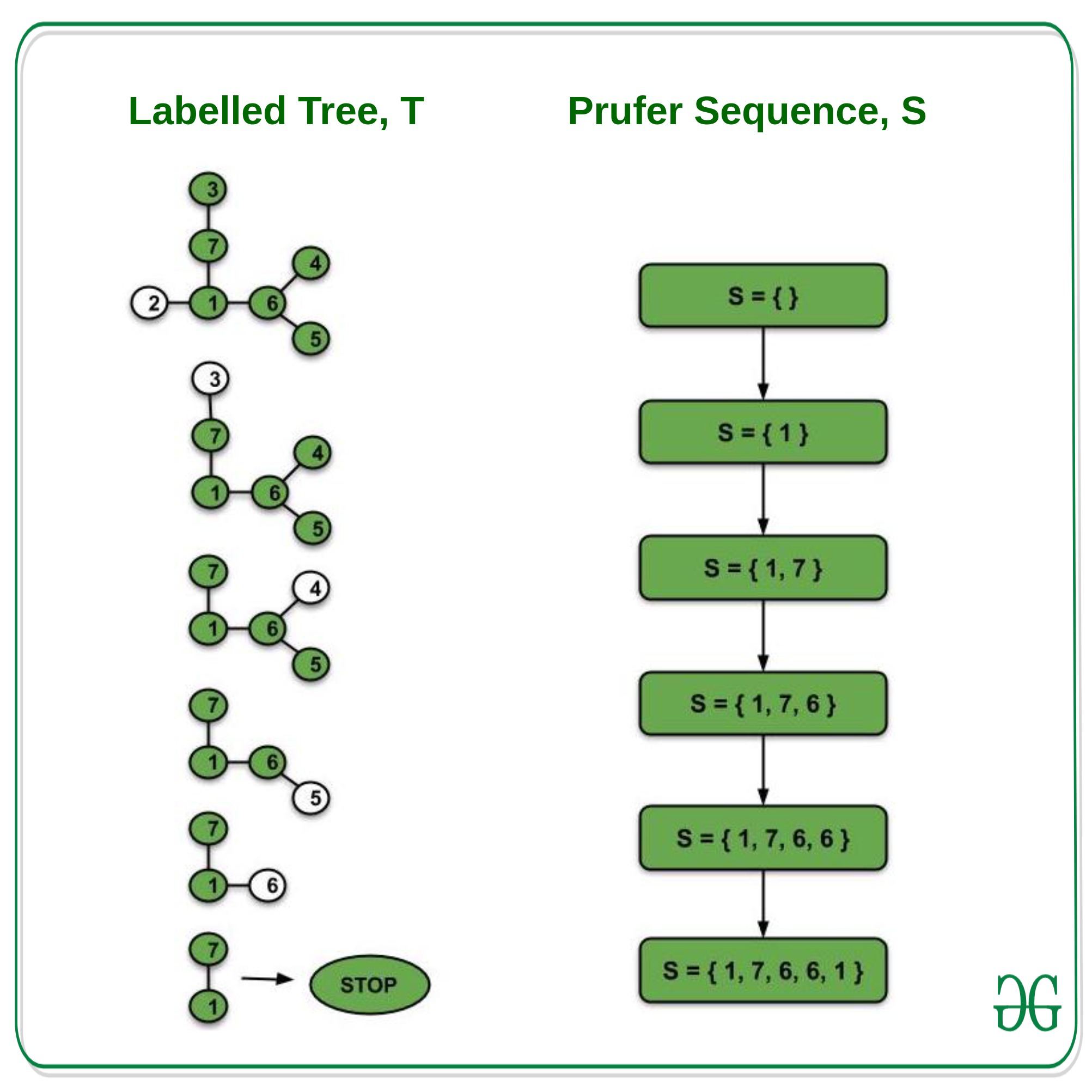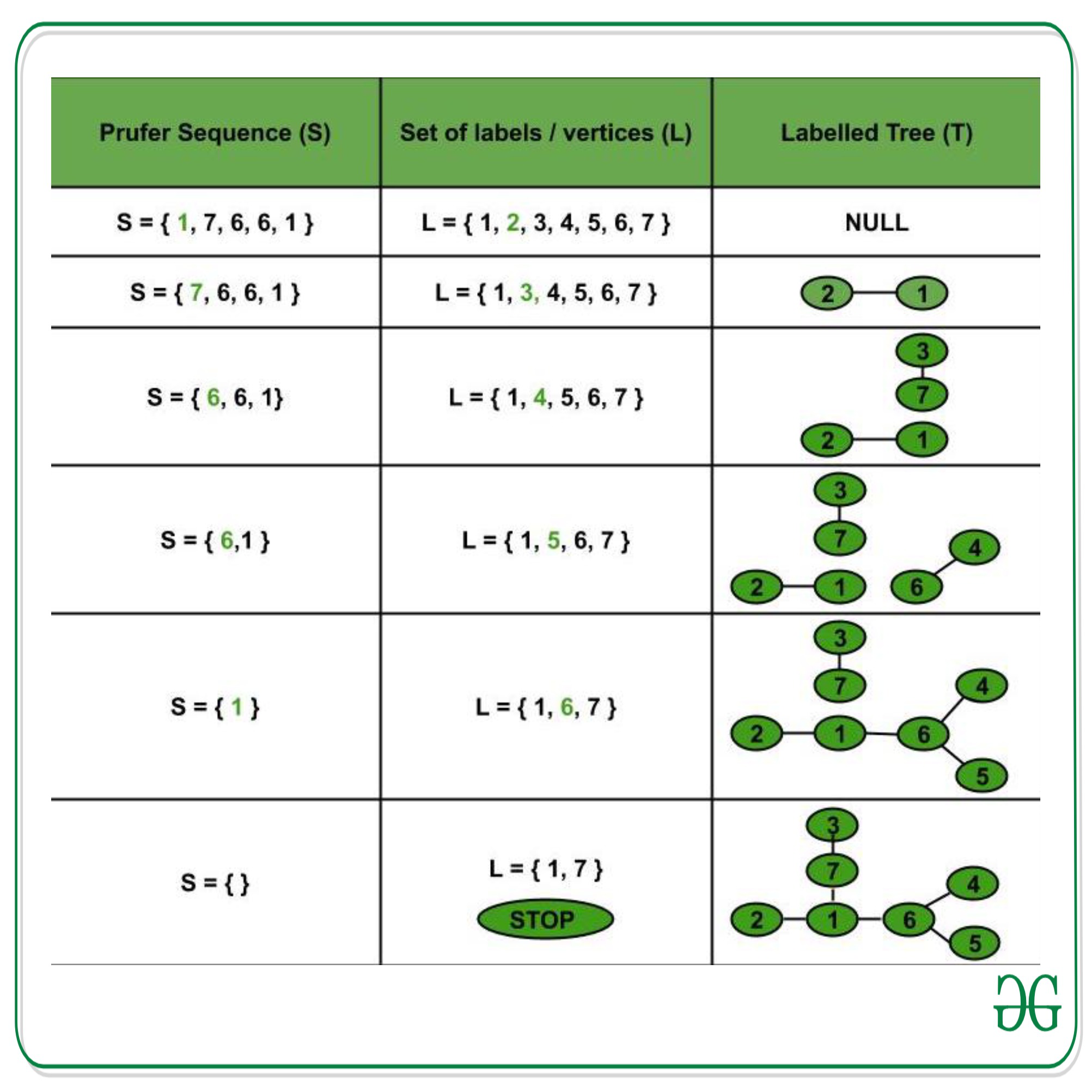# Number of spanning trees of a weighted complete Graph

Prerequisites: Graph Theory Basics, Spanning tree.

Complete Weighted Graph: A graph in which an edge connects each pair of graph vertices and each edge has a weight associated with it is known as a complete weighted graph.

The number of spanning trees for a complete weighted graph with n vertices is n(n-2).

Proof: Spanning tree is the subgraph of graph G that contains all the vertices of the graph. Therefore, the number of spanning trees of a complete weighted graph is the same as the number of labeled trees (need not be binary) with n vertices.

The Prüfer sequence of a labeled tree of n vertices is a unique sequence of length (n-2) associated with the tree. Also, for a given Prüfer sequence of length (n-2) on the labels 1 to n, there is a unique labeled tree with the given Prüfer sequence. Therefore, we have a bijection between the set A of labeled trees with n vertices and the set B of Prüfer sequences of size n-2 on the labels 1 to n. This can be proved as follows –

Let T be a labeled tree with vertices 1,2,…,n, and S as a Prüfer sequence of size (n-2). Thus, T and S are the elements of sets A and B, respectively.

(i) Labeled tree (T) –> Prufer sequence (S)

Constructing the Prüfer sequence of a labeled tree –

Initially, let S = NULL.

Procedure –

• Find the leaf node(L) of T with the smallest label.
• Add the neighbour of L to S.
• Delete the leaf node, L.
• Repeat the above steps until there are only two nodes left in the tree (Only one spanning tree is possible).
• We constructed the Prüfer sequence S associated with the labeled tree T.Observations –

• No leaf node is appended to S.
• Every vertex V of tree T is added to S, a total of degree(V)-1 times.
• The tree T has n vertices and hence (n-1) edges.
• Number of terms in S = Sum of (degree(V) – 1) for all vertices V belonging to the tree T = Sum of degree of all vertices of tree T – (1+1+…+1..n times) = 2(no. of edges) – n = 2*(n-1) – n = n-2. (Since the sum of the degree of all vertices of a tree = 2*no. of edges of the tree).
• Therefore, T is analogous to the Prüfer’s sequence S of length (n-2).

(ii) Prufer Sequence (S) –> Labeled Tree (T)

Constructing the labeled tree from its Prüfer sequence

Procedure-

• Let L = {1, 2, …, n} be the set of labels (vertices of T).
• Let S = {a1,a2,…,a(n-2)} be the Prüfer sequence of size (n-2) where each ai belongs to L.
• Find the smallest element x that belongs to L but is not in S.
• Connect x and the first element of S (a1) through an edge.
• Delete a1 from S, x from L (Thus, S:=S-{a1} and, L:=L-{x}).
• Similarly, find y, the smallest element belonging to L and not in S.
• Connect y and first element of S(a2).
• Remove y from L and a2 from S (Thus, S:=S-{a2} and, L:=L-{y}).
• Continue the above process till two items are left in L.
• Connect these two items in the tree formed so far.The tree obtained from S is the same as T. Therefore, Prüfer sequence S of size (n-2) is analogous to T ( S <–> T ). Hence, there is a bijection between the set of labeled trees with n vertices and the set of Prüfer sequences of size (n-2) on the labels 1 to n.

Thus, the number of spanning trees of a complete weighted graph of n vertices = number of labeled trees with n vertices = number of Prüfer sequences of size (n-2) = n(n-2).

Attention reader! Don’t stop learning now. Get hold of all the important DSA concepts with the DSA Self Paced Course at a student-friendly price and become industry ready.

My Personal Notes arrow_drop_upCheck out this Author's contributed articles.

If you like GeeksforGeeks and would like to contribute, you can also write an article using contribute.geeksforgeeks.org or mail your article to contribute@geeksforgeeks.org. See your article appearing on the GeeksforGeeks main page and help other Geeks.

Please Improve this article if you find anything incorrect by clicking on the "Improve Article" button below.